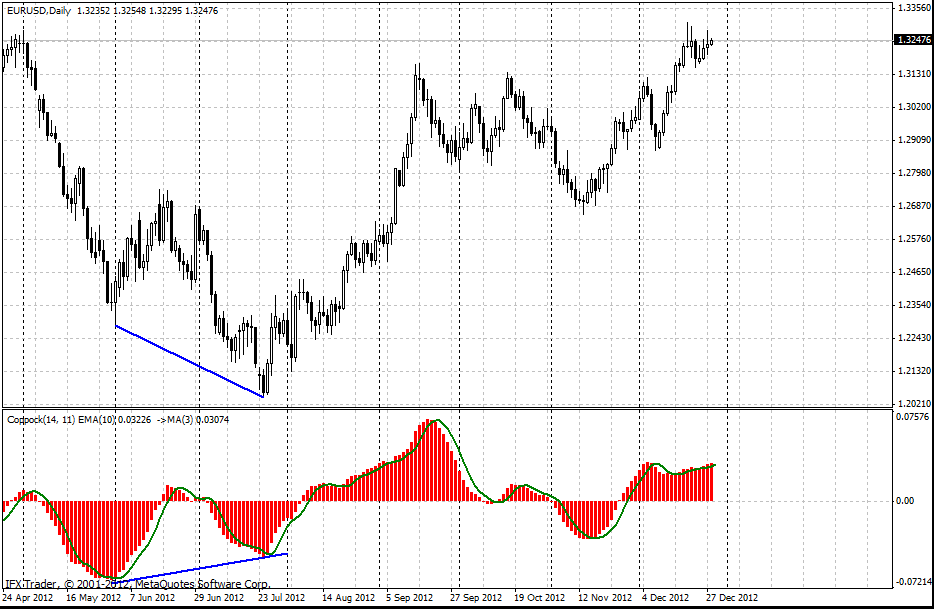#### Formula

Coppock(t) = EMA(w,t)[ROC(l,t) + ROC(s,t)], where

EMA(w,t) — exponential moving average on w periods;

[ROC(l,t) + ROC(s,t)] — sum of indicators of the speed the price changs for l (long) and s (short) periods.

According to the original approach, Coppock Indicator is calculated as a 10-month weighted moving average of the sum of the 14-month rate of change and the 11-month rate of change for the index. The signals of the indicator are observed when bar chart changes its direction, swinging up or down.

One more interesting application of Coppock indicator for MetaTrader is trading when level 0 is crossed. If a bar graph crosses the level from the bottom up, one should open buy deals and vice versa.

Robert W. Colby, a well-known technical analyst and the author of the book The Encyclopedia Of Technical Market Indicators recommended using Coppock indicator with 5-day exponential moving average.

When EMA leaves bar graph moving downwards, one should sell asset. And vice versa, when EMA leaves bar chart moving upwards, one should buy. This approach allows the traders to see the local extremum.#### Parameters of InstaForex Coppock indicator

ROC1Period = 14

ROC2Period = 11

MAPeriod = 10

MAType = 1

Can't speak right now?